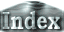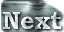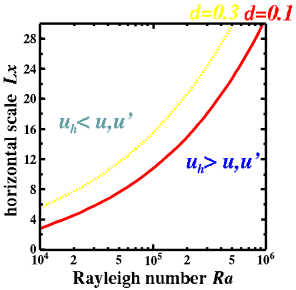Horizontal scales of convection cells (3) -Comparison of convective velocitiesFigure 10 compares velocity amplitudes with respect to Rayleigh number and the horizontal scale of the convection cell. The region below the line corresponds to uh >u,u'. Under these conditions we interpret the convection induced by the horizontal temperature difference to overcome the intrinsic convection induced by the vertical temperature difference, and convection cells with corresponding horizontal scales Lx can emerge. On the other hand, the region above the line corresponds to uh u,u' is found to be Lx=2 in the case of Ra=104, Lx=10 in the case of Ra=105, and Lx=30 in the case of Ra=106. In other words, convection cells whose aspect ratios are less than 2, 10 and 30 can emerge in the cases of Ra=104, 105 and 106, respectively. By substituting the horizontal size of the plate for Lx, we can determine whether convection cells of similar horizontal scales to the plate can emerge or not. When the plate width is located below the curve, a convection cell of the same horizontal scale as the plateˇÇs can emerge. Conversely, when the plate width plots above the curve, the horizontal scale of a convection cell will be less than that of the plate, as its maximum horizontal scale is limited by the line. In this case, the upwelling will emerge near the margins of the plate rather than at its center.Figure 10: The maximum aspect ratio of the convection cells. For given values of the Rayleigh number, convection cells whose horizontal sizes are smaller than the values indicated by the line can overcome the intrinsic convection induced by the vertical temperature difference. a=1.5 is adopted for the estimation using equation (10).

 Horizontal scales of convection cells (3) -Comparison of convective velocities